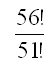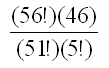From: Harvey Is it best to use factorials to calculate the odds of winning a lottery, such as the MegaMillions that is popular in the US, or is there a better way? Hi Harvey. Factorials are the way to go. The MegaMillions lottery requires a customer to choose any five distinct numbers between 1 and 56 as well as a single number between 1 and 46. This means that for the first number, you have 56 choices. For the second number, you have 55 choices (you can re-use your number), for the third, 54 choices, etc. We can write this as a factorial:The last number doesn't depend on the others; there are 46 possible choices for it. So we can just multiply by 46 to take care of that. Now remember that the last number (the one selected from 1-46) is identified separately from the others, but the first five are just a group of numbers where order doesn't matter. So we need to divide our answer so far by the number of ways you can re-organize the same 5 numbers. That's also a factorial: 5! When we put all this together, we get the final answer for the odds of winning the jackpot:If you do the calculation correctly, you'll get the same odds that MegaMillions reports. Stephen La Rocque.